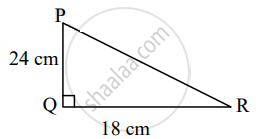# Height and base of a right angled triangle are 24 cm and 18 cm. Find the length of its hypotenus? - Geometry

Sum

Height and base of a right angled triangle are 24 cm and 18 cm. Find the length of its hypotenus?

#### SolutionIn ∆PQR, ∠Q = 90°

∴ PR2 = PQ2 + QR2      ......[Pythagoras theorem]

= 242 + 182

= 576 + 324

= 900

∴ PR = sqrt(900)

= 30 cm

Concept: Apollonius Theorem
Is there an error in this question or solution?

Share